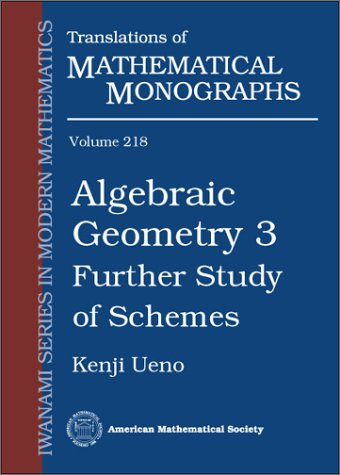## Series Monográficas: Translation of mathematical monographsVol. 197. Ueno, Kenji

QA564 U3513

Vol. 172. Berkovich, IA. G.; Zhmud, E. M.
Characters of finite groups
QA177 B4713

Vol. 171. Landis, Evgenii Mikhailovich
Second order equations of elliptic and parabolic type
QA377 L3513

Vol. 195. Wakimoto, Minoru
Infinite-dimensional Lie algebras
QA252.3 W3313

Vol. 170. Prasolov, Viktor Vasilevich; Solovyev, Yuri Pavlovich
Elliptic functions and elliptic integrals
QA343 P73

Vol. 194. Nevzorov, Valery B.
Records : mathematical theory
QA278.7 N4813

Vol. 169. Godunov, Sergei Konstantinovich
Ordinary differential equations with constant coefficient
QA372 G6313 1997

Vol. 193. Nishino, Toshio
Function theory in several complex variables
QA331.7 N5713

Vol. 168. Noguchi, Junjiro
Introduction to complex analysis
QA331.7 N6413

Vol. 192. Solovyev, Yuri Pavlovich
C*-algebras and elliptic operators in differential topology
QA326 S65513

Vol. 167. Yamaguchi, Masaya; Hata, Masayoshi; Kigami, Jun
Mathematics of fractals
QA614.86 Y3513 1997

Vol. 191. Amari, Shunichi; Nagaoka, Hiroshi

QA276 A56213

Vol. 166. Ueno, Kenji
An introduction to algebraic geometry
QA564 U45513 1997

Vol. 190. Starkov, Alexander N.
Dynamical systems on homogeneous spaces
QA614.82 S7313

The embedding problem in Galois theory
QA214 I7413 1997

Vol. 164. Gordon, Evgenii Izrailevich
Nonstandard methods in commutative harmonic analysis
QA403 G67 1997

Vol. 188. Buldygin, Valerii Vladimirovich; Kozachenko

QA273 B8313

Vol. 163. Dorogovtsev, A. Ya; Silvestrov, D. S.; Skorokhod,A. V.; Yadrenko, M. I.
Probability Theory: Collection of Problems
QA273.25 T4613 1997

Vol. 187. Fursikov, A. V.;
Optimal control of distributed systems : theory and applications
QA402.3 F8713

Vol. 162. Boldin, M. V.; Simonova, Galina I.; Tyurin, Yu Nikolaevich
Sign-based methods in linear statistical models
QA279 B64 1997

Vol. 186. Kato, Kazuya
Number theory 1: Fermat's Dream
QA241 K37413

Vol. 161. Blank, Michael L.
Discreteness and continuity in problems of chaotic dynamics
QA611.5 B53 1997

Vol. 160. Osmolovskii, Viktor Grigorevich
Linear and nonlinear perturbations of the operator div
QA379 O7513 1997

Vol. 183. Sato, Hajime
Algebraic topology : an intuitive approach
QA612 S35513

Vol. 158. Omori, Hideki
Infinite-dimensional Lie groups
QA613.2 O5613 1997

Vol. 182. Krasil’shchik, Iosif Semenovich; Vinogradov, Aleksandr Mikhailovich (Eds.)
Symmetries and conservation laws for differential equations of mathematical physics
QC20.7D5 S54913

Vol. 157. Kolmanovskii, Vladimir Borisovich; Shaikhet, Leonid Efimovich
Control of systems with aftereffect
QA402.3 K6513 1996

Vol. 181. Berkovich, IA. G.; Zhmud, E. M.
Characters of finite groups. Part II
QA177 B4713

Vol. 156. Shevchenko, Valery N.
Qualitative topics in integer linear programming
T57.74 S45513 1997

Vol. 180. Miliutin, A. A.; Osmolovskii, Nikolai Pavlovich
Calculus of variations and optimal control
QA315 M5513

Vol. 155. Safarov, Yuri; Vassilev, D.
The asymptotic distribution of eigenvalues of partial differential operators
QA329.42 S34 1997

Vol. 179. Voskresenskii, Valentin Evgen'evich
Algebraic groups and their birational invariants
QA179 V6713

Vol. 154. Prasolov, Viktor Vasilevich; Sossonsky, A. B.
Knots, links, braids and 3-manifolds : an introduction to the new invariants in low-dimensional topology
QA612.14 P73

Vol. 178. Morimoto, Mitsuo
Analytic functionals on the sphere
QA331 M668

Vol. 152. Ismagilov, Rais Salmanovich
Representations of infinite-dimensional groups
QA387 I75 1996

Vol. 176. Lerman, Lev M.; Umanskiy, Ya L.

QA614.83 L47

Vol. 151. Slavianov, Sergei IUrevich
Asymptotic solutions of the one-dimensional Schrödinger equation
QC174.26W28 S55513 1996

Vol. 175. Godunov, Sergei Konstantinovich
Modern aspects of linear algebra
QA184 .G5813

Vol. 150. Levin, Boris IAkovlevich
Lectures on entire functions
QA351.E5 L4813 1996

Vol. 174. Chen, Yazhe; Wu, Lancheng
Second order elliptic equations and elliptic systems
QA377 C4413

Vol. 149 Sakai, Takashi
Riemannian geometry
QA649 S33713

Vol. 173. Davydov, Iurii Aleksandrovich; Lifshietis, Mikhail Anatolevich; Smorodina, Nataleia Vasilevna
Local properties of distributions of stochastic functionals
QA273.67 D3813